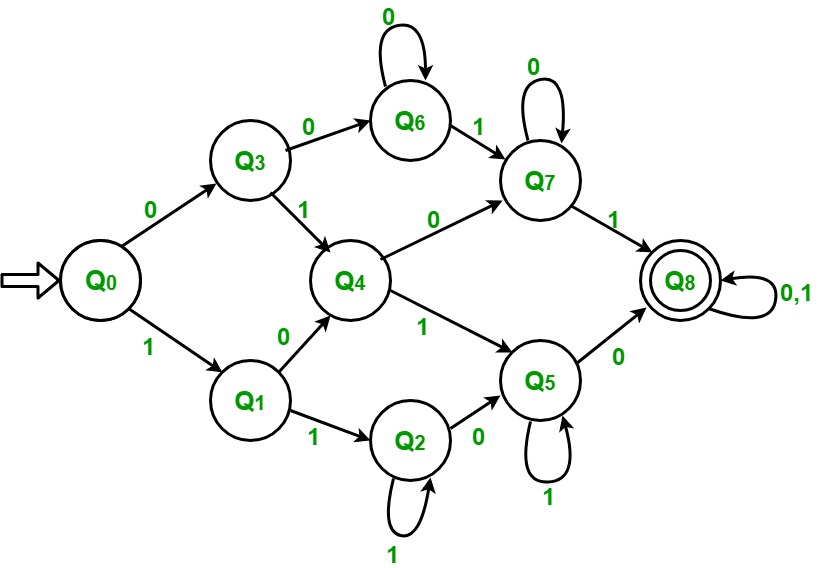# DFA of a string with at least two 0’s and at least two 1’s

Problem – Draw detereministic finite automata (DFA) of a string with at least two 0’s and at least two 1’s.

The first thing that come to mind after reading this question us that we count the number of 1’s and 0’s. Thereafter if they both are at least 2 the string is accepted else not accepted. But we do not have any concept of memory in a DFA so we cannot do it by this method.

```Input  : 1 0 1 1 0 0
Output : Accepted

Input  : 1 1 1 0 1
Output : Not accepted
```

Approach Used –
The first thing we observe is that both 0’s and 1’s should be at least 2. If any of these is less than 2, then string will not be accepted. In this string will be accepted in only last case where both 0’s and 1’s will be at least 2.

State Count of 0 Count of 1
Q0 0 0
Q1 0 1
Q2 0 >=2
Q3 1 0
Q4 1 1
Q5 1 >=2
Q6 >=2 0
Q7 >=2 1
Q8 ACCEPTED >=2 >=2

Initially count of both 0 and 1 is zero and we are on state Q0.

• Step-1: If input is 1 then count of 1 increases to 1. Goto state Q1
If input is 0 then count of 0 increases to 1. Goto state Q3
• Step-2: If input is 1 then count of 1 increases to 2. Goto state Q2
If input is 0 then count of 0 increases to 1. Goto state Q4

• Step-3: If input is 1 then count of 1 keeps increasing by 1. Remain in the same state
If input is 0 then count of 0 increases to 1. Goto state Q5

• Step-4: If input is 1 then count of 1 increases to 1. Goto state Q4
If input is 0 then count of 0 increases to 2. Goto state Q6

• Step-5: If input is 1 then count of 1 increases to 2. Goto state Q5
If input is 0 then count of 0 increases to 2. Goto state Q7

• Step-6: If input is 1 then count of 1 keeps increasing by 1. Remain in the same state.
If input is 0 then count of 0 increases to 2. Goto state Q8

• Step-7: If input is 1 then count of 1 increases to 1. Goto state Q7
If input is 0 then count of 0 keeps increasing by 1. Remain in the same state.

• Step-8: If input is 1 then count of 1 increases to 2. Goto state Q8
If input is 0 then count of 0 keeps increasing by 1. Remain in the same state.

• Step-9: If input is 1 then count of 1 keeps increasing by 1. Remain in the same state.
If input is 0 then count of 0 keeps increasing by 1. Remain in the same state.
If string is finished then ACCEPTEDGeeksforGeeks has prepared a complete interview preparation course with premium videos, theory, practice problems, TA support and many more features. Please refer Placement 100 for details

My Personal Notes arrow_drop_upCheck out this Author's contributed articles.

If you like GeeksforGeeks and would like to contribute, you can also write an article using contribute.geeksforgeeks.org or mail your article to contribute@geeksforgeeks.org. See your article appearing on the GeeksforGeeks main page and help other Geeks.

Please Improve this article if you find anything incorrect by clicking on the "Improve Article" button below.

Article Tags :

3

Please write to us at contribute@geeksforgeeks.org to report any issue with the above content.